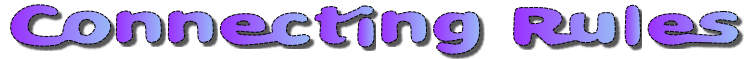Here are some equations that are true when x=3 and y=4 but there is at least one mistake. Click on the ticks and crosses to show this piece of work correctly marked.

 x + y = 7x - y = -1y - x = 12x + 3y = 225x - 2y = 74y - x = 13xy - 10 = 2180 - 2x + y = 17820x - 3xy = 24x2 + y = 2310x - y2 = 25y2 - x2 = 7x2y + 13 = 62x2y2 - 2x = 138x2y3 = 576x! + y = 105(x + y) = 353y ÷ x = 4xy 2 = 684 xy = 736y 5x = yTransum.org

This web site contains over a thousand free mathematical activities for teachers and pupils. Click here to go to the main page which links to all of the resources available.

Please contact me if you have any suggestions or questions.More Activities:

Mathematicians are not the people who find Maths easy; they are the people who enjoy how mystifying, puzzling and hard it is. Are you a mathematician?

Comment recorded on the 3 October 'Starter of the Day' page by Fiona Bray, Cams Hill School:

"This is an excellent website. We all often use the starters as the pupils come in the door and get settled as we take the register."

Comment recorded on the 17 June 'Starter of the Day' page by Mr Hall, Light Hall School, Solihull:

"Dear Transum,

I love you website I use it every maths lesson I have with every year group! I don't know were I would turn to with out you!"

Roman Numerals JigsawThis is a wonderful activity for someone who does not know Roman numerals. By completing the activity an understanding of the symbols develops and a great sense of achievement is enjoyed.

Numeracy

"Numeracy is a proficiency which is developed mainly in Mathematics but also in other subjects. It is more than an ability to do basic arithmetic. It involves developing confidence and competence with numbers and measures. It requires understanding of the number system, a repertoire of mathematical techniques, and an inclination and ability to solve quantitative or spatial problems in a range of contexts. Numeracy also demands understanding of the ways in which data are gathered by counting and measuring, and presented in graphs, diagrams, charts and tables."

Secondary National Strategy, Mathematics at key stage 3

Go Maths

Learning and understanding Mathematics, at every level, requires learner engagement. Mathematics is not a spectator sport. Sometimes traditional teaching fails to actively involve students. One way to address the problem is through the use of interactive activities and this web site provides many of those. The Go Maths main page links to more activities designed for students in upper Secondary/High school.

Do you have any comments? It is always useful to receive feedback and helps make this free resource even more useful for those learning Mathematics anywhere in the world. Click here to enter your comments.For All: